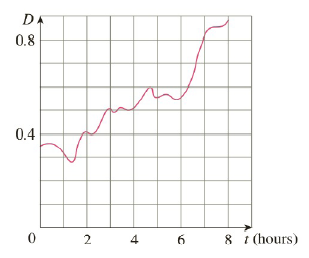Chapter 4.4, Problem 66E

Chapter
Section
Textbook Problem

# Shown is the graph of traffic on an Internet service provider’s T1 data line from midnight to 8:00 am. D is the data throughput, measured in megabits per second. Use the Midpoint Rule to estimate the total amount of data transmitted during that time period.To determine

To estimate:

The total amount of data transmitted during time period midnight to 8:00 A.M.

Explanation

1) Concept:

Use the Net change theorem and midpoint rule.

2) Theorem and Rule:

The Net Change theorem: The integral of a rate of change is the net change.

abF'(x)dx=Fb-F(a)

The Midpoint rule:

abfxdxi=1nfxi- x=x fx1-++fxn-

where x=b-an and xi-=12xi-1+xi midpoint of xi-1, xi.

3) Calculation:

Let Mt denote the number of megabits transmitted at the time t(in hours).

Dt  measured in megabits/second is the rate of change of number of megabits transmitted at time t.

Dt=M'(t)

Here,t0,8

By the Net Change theorem,

08Dt dt08M'tdt=M8-M(0) is number of megabits transmitted during the time interval.

Approximate the value of the integral using the Midpoint Rule with n=4, a=0, and b=8

### Still sussing out bartleby?

Check out a sample textbook solution.

See a sample solution

#### The Solution to Your Study Problems

Bartleby provides explanations to thousands of textbook problems written by our experts, many with advanced degrees!

Get Started

#### Find more solutions based on key concepts